﻿ Influence of the Density of Water on the Dynamic Behavior of Square Tension Leg Platform

### Influence of the Density of Water on the Dynamic Behavior of Square Tension Leg Platform

Hala M. Refat, Amr R. El-gamalOPEN ACCESSPEER-REVIEWED

## Influence of the Density of Water on the Dynamic Behavior of Square Tension Leg Platform

Hala M. Refat1,, Amr R. El-gamal1

1Civil Engineering Department, Faculty of Engineering at Benha, Benha University, Egypt

### Abstract

tension-leg platform (TLP) or extended tension leg platform (ETLP) is a vertically normally used for the offshore production of or , and is particularly suited for water depths greater than 300 meters and less than 1500 meters. The platform is permanently moored by means of tethers or tendons grouped at each of the structure's corners. A group of tethers is called a tension leg. A feature of the design of the tethers is that they have relatively high (low ), such that virtually all vertical motion of the platform is eliminated. This allows the platform to have the production on deck (connected directly to the subsea wells by rigid risers), instead of on the . This allows a simpler and gives better control over the production from the or , and easier access for down whole intervention operations. In this paper a numerical study for a square TLP using modified Morison equation was carried out in the time domain with water particle kinematics using Airy’s linear wave theory to investigate the effect of changing water density on the mass matrix of TLP's and the dynamic behavior of TLP's. The effect was investigated for different parameters of the hydrodynamic forces such as wave periods, and wave heights. The numerical study takes into consideration the effect of coupling between various degrees of freedom. The stiffness of the TLP was derived from a combination of hydrostatic restoring forces and restoring forces due to cables. Nonlinear equation was solved using Newmark’s beta integration method. Only uni-directional waves in the surge direction was considered in the analysis.

### At a glance: Figures

• Refat, Hala M., and Amr R. El-gamal. "Influence of the Density of Water on the Dynamic Behavior of Square Tension Leg Platform." American Journal of Civil Engineering and Architecture 2.4 (2014): 122-129.
• Refat, H. M. , & El-gamal, A. R. (2014). Influence of the Density of Water on the Dynamic Behavior of Square Tension Leg Platform. American Journal of Civil Engineering and Architecture, 2(4), 122-129.
• Refat, Hala M., and Amr R. El-gamal. "Influence of the Density of Water on the Dynamic Behavior of Square Tension Leg Platform." American Journal of Civil Engineering and Architecture 2, no. 4 (2014): 122-129.

 Import into BibTeX Import into EndNote Import into RefMan Import into RefWorks

### 1. Introduction

A type of floating production system, tension leg platforms (TLPs) are buoyant production facilities vertically moored to the seafloor by tendons. While a buoyant hull supports the platform's topsides, an intricate mooring system keeps the TLP in place. The buoyancy of the facility's hull offsets the weight of the platform, requiring clusters of tight tendons, or tension legs, to secure the structure to the foundation on the seabed. The foundation is then kept stationary by piles driven into the seabed. The tension leg mooring system allows for horizontal movement with wave disturbances, but does not permit vertical, or bobbing, movement, which makes TLPs a popular choice for stability, such as in the hurricane-prone Gulf of Mexico. The basic design of a TLP includes four air-filled columns forming a square. These columns are supported and connected by pontoons, similar to the design of a semisubmersible production platform. Nonetheless, since their inception in the mid 1980s, TLP designs have changed according to development requirements. Now, designs also comprise the E-TLP, which includes a ring pontoon connecting the four air-filled columns; the Moses TLP, which centralizes the four-column hull; and the Sea Star TLP, which includes only one central column for a hull. The platform deck is located atop the hull of the TLP. The topside of a TLP is the same as a typical production platform, consisting of a deck that houses the drilling and production equipment, as well as the power module and the living quarters. Dry tree wells are common on TLPs because of the lessened vertical movement on the platforms. Most wells producing to TLPs are developed through rigid risers, which lift the hydrocarbons from the seafloor to dry trees located on the TLP deck. Many times, steel catenaries risers are also used to tie-in the subsea flow lines and export pipelines. The third-most used type of floating production facility in the world, TLPs are ideal for a broad range of water depths. Currently, there are three different types of TLPs: full-size TLPs, mini TLPs and wellhead TLPs.

Many papers available as, Paulling and Horton  reported a method of predicting the platform motions and tether forces due to regular waves using a linearized hydrodynamic synthesis technique. Each member was assumed to be cylindrical in shape with cross-sectional dimensions small in comparison to both the length of the cylinder and the wave length. Ahmad et al,  studied the effect of the variable submergence on the maximum tether tension force on TLP with change in wave incidence angle. The study was carried out on coupled and uncoupled model. Jain  dynamic response analysis of a TLP to deterministic first order wave forces is presented, considering coupling between the degrees-of-freedom (surge, sway, heave, roll, pitch and yaw). The analysis considers nonlinearities produced due to changes in cable tension and due to nonlinear hydrodynamic drag forces. The wave forces on the elements of the pontoon structure are calculated using Airy's wave theory and Morison's equation ignoring diffraction effects. The nonlinear equation of motion is solved in the time domain by Newmark's beta integration scheme. The effects of different parameters that influence the response of the TLP are then investigated. Like change in tether tension force and damping ratio. Chan K. Yang, M. H. Kim  developed a numerical study of the transient effect of tendon disconnection on global performance of an extended tension leg platform (ETLP) during harsh environmental conditions of Gulf Of Mexico (GOM). Kurian et al.  developed a numerical study on the dynamic response of square TLPs subjected to regular and random waves. They also conducted parametric studies with varying parameters such as water depth, pretension, wave angle and position of center of gravity. Low  presented a formulation for the linearization of the tendon restoring forces of a TLP. , , , , study reliability assessment of Tension Leg Platform (TLP) tethers against maximum tension (i.e. tension exceeding yield) has been carried out under combined action of extreme wave and impulsive forces. Xiaohui Zeng, Yang Liu, Xiaopeng Shen, Yingxiang Wu , study the dynamic responses of TLP with finite displacements. Amir Hossein Razaghian, Mohammad Saeid Seif, Mohammad Reza Tabeshpour , evaluates the hydrodynamic performance of a damaged ISSC TLP which is caused by a tendon disconnection. , , Study the solution for the Duffing equation in a nonlinear vibration problem. A.M.Abou-Rayan, Ayman A. Seleemah, and Amr R. El-gamal  developed a numerical study on determining the dynamic responses of TLPs subjected to regular wave. They found that coupling between various degrees of freedom has insignificantly dependent on the wave height; whereas for longer wave periods of 15 sec.

In this paper, a numerical study was conducted to investigate the dynamic response of a square TLP (shown in Figure 2) under hydrodynamic forces considering all degrees of freedom of the system (shown in Figure 1). The analysis was carried out using modified Morison equation in the time domain with water particle kinematics using Airy’s linear wave theory to investigate the effect of changing the water density on the mass matrix of TLP's and the dynamic behavior of TLP's.. The effect was investigated for different parameters of the hydrodynamic forces such as wave periods, and wave heights. The numerical study takes into consideration the effect of coupling between various degrees of freedom. The stiffness of the TLP was derived from a combination of hydrostatic restoring forces and restoring forces due to cables and the nonlinear equations of motion were solved utilizing Newmark’s beta integration scheme. The effect of wave characteristics such as wave period and wave height on the response of TLP's was evaluated. Only uni-directional waves in the surge direction was considered in the analysis.

### 2. Structural Idealization and Assumptions

The general equation of motion of the square configuration TLP model under a regular wave is given as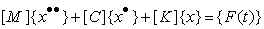(1)

where, {x} is the structural displacement vector, {x˙} is the structural velocity vector, {x¨} is the structural acceleration vector; [M] is the structure mass matrix; [C] is the structure damping matrix; [K] is the structure stiffness matrix; and {F(t)} is the hydrodynamic force vector.

The mathematical model derived in this study assumes that the platform and the tethers are treated as a single system and the analysis is carried out for the six degrees of freedom under different environmental loads where wave forces are estimated at the instantaneous equilibrium position of the platform utilizing Morison’s equation and using Airy’s linear wave theory. Wave force coefficients, Cd and Cm, are the same for the pontoons and the columns and are independent of frequencies as well as constant over the water depth. The following assumptions were made in the analysis.

1. Change in pre-tension is calculated at each time step, so the equation of equilibrium at each time step modifies the elements of the stiffness matrix.

2. The platform has been considered symmetrical along the surge axis. Directionality of wave approach to the structure has been ignored in the analysis and only a uni-directional wave train has been considered.

3. The damping matrix has been assumed to be mass and stiffness proportional.

4. The force on tethers (gravity, inertia, and drag, hydrostatic and hydrodynamic forces) has been neglected because of its small area and also the tether curvature is not significant in motion; only the axial forces acting on tethers have been considered.

5. Hydrodynamic forces on connecting members and mooring legs have been neglected.

6. The wave, current and structure motions are taken to occur in the same plane and in the same direction, the interaction of wave and current has been ignored.

7. Integration of hydrodynamic inertia and drag forces are carried out up to the actual level of submergence, when variable submergence is considered.

### 3. Development of a Square TLP Model

3.1. Draft Evaluation

At the original equilibrium position, Figure 3, summation of forces in the vertical direction gives: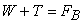(2)

We find that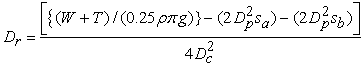(3)

where, FB is the total buoyancy force; W is the total weight of the platform in air; T is the total instantaneous tension in the tethers; To is the initial pre-tension in the tether; p is the mass density of sea water; Dc is the diameter of TLP columns; Dp is the diameter of pontoon; Sa and Sb are the length of the pontoon between the inner edges of the columns in the x and y directions, respectively; and Dr is the draft.

We notice from eq. (3) that drift distance inversely proportion to water density.

3.2. Stiffness Matrix of Square TLP Configuration

The stiffness of the platform is derived from a combination of hydrostatic restoring forces and restoring forces due to the cables. Restoring force for motions in the horizontal plane (surge, sway, and yaw) are the horizontal component of the pretension in the cables, while restoring forces for motions in the vertical plane arise primarily from the elastic properties of the cables, with a relatively small contribution due to hydrostatic forces.

For more detailed about the derivation of the stiffness matrix, the reader is referred to .

The overall stiffness matrix shows:

1. The presence of off-diagonal terms, which reflects the coupling effect between the various degrees of freedom.

2. The coefficients depend on the change in the tension of the tethers, which is affecting the buoyancy of the system. Hence, the matrix is response dependent.

3. Hence, during the dynamic analysis, the [K] matrix is not constant for all time instants, but its components are continuously changing at each time step depending upon the response values at the previous time step.

4. The coefficient for heave stiffness matrix depends on water density while the surge coefficient directly doesn’t depend on the water density.

3.3. Mass Matrix, [M]

The mass matrix is assumed to be lumped at each degree of freedom. Hence, it is diagonal in nature and is constant. However, the added mass, Ma, due to the water surrounding the structural members has been considered up to the mean sea level (MSL) and arising from the modified Morison equation. The presence of off diagonal terms in the mass matrix indicates a contribution of the added mass due to the hydrodynamic loading. The fluctuating components of added mass due to the variable submergence of the structure in water is considered in the force vector depending upon whether the sea surface elevation is above or below the MSL. The loading will be attracted only in the surge, heave and pitch degrees of freedom due to the unidirectional wave acting in the surge direction on a symmetric configuration of the platform about the x and z axes.

For more detailed about the derivation of the mass matrix, the reader is referred to .

3.4. Structural Damping [C]

Structure Damping was presented in the form of mass matrix is assumed to be mass and restoring force proportional,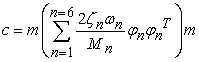(4)

Where; {Øn} and ωn are the mode shapes and structure's natural frequencies, ζ n is the structural damping ratio, C is the damping matrix, m is the total structure mass matrix and Mn is the corresponding element of the { Øn}T [m]{ Øn}

This matrix is calculated based on the initial values of [K] and [M] depending on the type of the platform.

3.5. Hydrodynamic Force Vector, {F(t)} on Square TLP

The hydrodynamic force vector is calculated in each degree of freedom according to modified Morison’s equation which takes into account the relative velocity and acceleration between the structure and the fluid particles. It is also worth mentioning that the ratio d/H can be related to d/λ. Based on the limiting heights of breaking waves, it become unstable and break when H/λ ≥ o.1, (H is the wave height and λ is the wave length).

For the uni-directional wave train in the surge direction, the force vector {F (t)}, is given by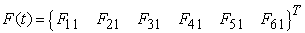(5)

Since the wave is unidirectional, there would be no force in the sway degree-of-freedom F21 and hence there will be no moment in the roll degree of-freedom F41. Because of the vertical water particle velocity and acceleration, the heave degree-of-freedom would experience wave force F31. The force in the surge direction F11 on the vertical members will cause moment in the pitch degree-of-freedom F51. However, forces in the surge degree-of-freedom are symmetrical about the X axis (due to the symmetry of the platform to the approaching wave) and there will be no net moment caused in the yaw degree-of-freedom F61.

For more detailed about the derivation of the force vector, the reader is referred to .

3.6. Solution of the Equation of Motion in the Time Domain

The equation of motion is coupled and nonlinear and can be written as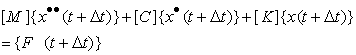(6)

Equation (6) is nonlinearly coupled, because of the presence of structural displacement, velocity and acceleration in the right hand side of the equation. Therefore, the force vector should be updated at each time step to account for the change in the tether tension. To achieve this response variation a time domain analysis is carried out. The Newmark's beta time integration procedure is used in a step wise manner. This procedure was developed by Newmark together with a family of time-stepping methods. The following values are updated

1. Stiffness coefficients which vary with tether pre-tension

2. Added mass which varies with sea surface fluctuations.

3. Wave forces at the instantaneous position of the displaced structure.

### 4. Results and Discussion

A numerical program was developed using MATLAB software where solution based on Newmark's beta method was obtained. The studied TLP geometrical, physical and the mechanical properties of the tethers are shown in Table 1.

#### Table 1. Geometric Properties of the Square TLP and Load Data

The main dynamic charchatrstic of the system chosen in the study are given in Table 2, Such as natural period of the square TLP.

#### Table 2. Calculated Natural Structural Periods for Different Analysis Cases (in Seconds)

It can be note from table II that square TLP has a high natural period in horizontal plan where as small natural period in vertical direction.

Also, it can be seen that changing the water density has a small effect on the natural period.

4.1. Surge Response

The time histories of the surge responses for the square TLP are shown in Figure 4 to Figure 7. It is observed that, for a specific wave period, the amplitude of oscillations increases as the wave height increases. For short period the system responds in small amplitude oscillations about a displaced position that is dependent to wave height and water density. The amplitude of oscillations increases with the increase in the wave period, which is expected because as the wave period increases, it becomes closer to the surge natural period of vibration (about 150 sec.). Moreover, in all cases, the surge response seems to have periodic oscillations that have the same exciting wave period.

The effect of water density is obvious in Figure 4 to Figure 7 which indicate that it affect the drift value of the displaced position. As water density becomes bigger the drift decreases but the water density has little effect on the amplitude of the oscillation. Finally, the transient state takes about 80-100 seconds where the stationary state begins.

It is obviously that Form Figure 4 - Figure 6 notice that the water density is inversely proportional to the wave period.

Drift distance is inversely proportional to the wave period

We notice that the water density increase the amplitude of surge response increase which lead to increase in tether in tension as when water density increase with 17.5% lead to increase in amplitude with 21 %.

We can see that the high wave period the change in water density has small effect.

4.2. Heave Response

The time histories are shown in Figs. 8 to 11. As expected, the response in the heave direction has very small values compared to that of the surge direction. This is attributed to the relatively high stiffness of the tethers in this direction together with the fact that the excitation is indirect in this case. Moreover, the heave response is inversely proportional to the wave period and directly proportional to wave height. The heave response appears to have a mean value of nearly zero. It is obvious that the increase of water density decrease slightly the amplitude of the heave response. Also, the transient state takes about 10-20 seconds where the stationary state begins and the motion is almost periodic.

The increase of water density with 17.5% leads to increase in heave response with 42.5%.

As wave period decrease the amplitude for heave response increase.

4.3. Pitch Response

The time histories shown in Figs. 12 to 15 it is clear that the increase of water density increase the amplitude of the pitch response and that effect is more obvious for small wave period with ratio of 50 %. Also the pitch response is inversely proportional to the wave period and directly proportional- but to a less extent- to wave height. The pitch response appears to have a mean value of nearly zero. Moreover, the transient state takes about 20-30 seconds before the stationary state begins.

As water density increase with 17.5% lead to increase in heave response with 50%.

4.4. Change in Tether Tension Force

The time histories for the change in tether tension force for the square TLP are shown in Figs. 16 to 19.

It is noted that the change in density lead to increase the tether tension with 15% of it is initial force which may lead to fatigue failure.

For long wave period the change in water density is not affected in the change in tether tension but the amplitude in tether tension force is big.

For short wave period it can be observed that the change in water density is affected in the change in tether tension but the amplitude of change is tether force is small

We notice that the change in tether tension has the same behavior of surge response which means that the surge movement has great effect on the tether tension force.

As water density increase with 17.5% leads to increase in change in tether tension with 27.5%.

Moreover, the transient state takes about 80-100 seconds before the stationary state begins.

Figure 19. Change in tether tension force of square TLP for Wave Height = 10 m and wave period = 10 sec

### 5. Conclusions

In this study a computer program was conducted to investigate the dynamic response of a square TLP under hydrodynamic forces considering all degrees of freedom of the system. The analysis was carried out using modified Morison equation in the time domain with water particle kinematics using Airy’s linear wave theory to investigate the effect of changing the water density on the mass matrix of TLP's and the dynamic behavior of TLP's. The effect was investigated for different parameters of the hydrodynamic forces such as wave periods, and wave heights. The numerical study takes into consideration the effect of coupling between various degrees of freedom. The stiffness of the TLP was derived from a combination of hydrostatic restoring forces and restoring forces due to cables and the nonlinear equations of motion were solved utilizing Newmark’s beta integration scheme. The effect of wave characteristics such as wave period and wave height on the response of TLP's was evaluated. Only uni-directional waves in the surge direction was considered in the analysis. The main conclusions were

1. The water density value effect the natural period for horizontal movement of square TLP while has little effect for vertical movements.

2. The water density value effect the amplitude of surge movement where, their effects on the amplitude of heave and pitch movements is less noticed.

3. The transition time for square TLP to reach a stable condition may reach to 100 second.

4. For same stress level in tethers the change in tether tension force leading to probability of fatigue failure is more pronounced in short move periods and in small value of tether pretension force.

5. Increase in density leads to increase in amplitude in response which has a great effect in tether tension force so we must replace the stiffness of tether with high density

6. Transit state in Surge response takes more time (100 sec) than heave and pitch response (20 sec)

7. Water density has a big effect in heave and pitch ratio with ratio 40: 50 %but in surge response has a little effect with ratio 17%.

### References

  Abou-Rayan, A.M., Seleemah, A., and El-gamal, A.R., "Response of Square Tension Leg Platforms to Hydrodynamic Forces", Ocean Systems Engineering, Vol. 2 (2), 2012, pp. 115-135.In article CrossRef  Ahmed, S., Bhaskar sengupta, A. Ali, "Nonlinear Dynamic Response of Tension Lrg Platform Tether under Offshore Environmental Conditions", Ocean Engineering, Vol.30, 1990, pp. 232-241.In article  Chan K. Yang, M. H. Kim, 2010, "Transient Effects of Tendon Disconnection of a TLP by Hull-Tendon –Riser Coupled Dynamic Analysis", Ocean Engineering, Vol. 37, 2010, pp. 667-677.In article CrossRef  Jain, A. K., "Nonlinear Coupled Response of Offshore Tension Leg Platforms to Regular Wave Forces", Ocean Engineering, Vol. 24, 1997, pp. 577-592.In article CrossRef  Pauling, J. R, Horton, E.E., "Analysis of the Tension Leg Stable Platform", In: Proceedings of the Offshore Technology Conference, OTC NO. 1263, 1970, pp. 379-390.In article  Kurian, V.J., Gasim, M.A., Narayanan, S.P., Kalaikumar, V., "Parametric Study of TLPs Subjected to Random Waves", ICCBT-C-19, pp. 213-222, 2008.In article  Low, Y. M., "Frequency domain analysis of a tension leg platform with statistical linearization of the tendon restoring forces", Marine Structures, Vol. 22, 2009, 480-503.In article CrossRef  Xiaohui Zeng, Yang Liu, Xiaopeng Shen, Yingxiang Wu, "Nonlinear Dynamic Response of Tension Leg Platform", In: proceeding of the Sixteenth International Offshore and Polar Engineering Conference San Francisco, California, USA, May 28-June 2, 2006, pp.94-100.In article  R.A. Khan, N.A. Siddiquia, S.Q.A. Naqvi, S. Ahmad," Reliability analysis of TLP tethers under impulsive loading", Reliability Engineering & System Safety, Volume 91, Issue 1, January 2006, Pages 73-83.In article CrossRef  Amir Hossein Razaghian, Mohammad Saeid Seif, Mohammad Reza Tabeshpour, "Investigation of tendons and TLP behavior in damaged condition ", International journal of maritime technology, Volume 9, Number 18 (5-2014). 23-34.In article  Rahim Shoghi, Mohammad Reza Tabeshpour," An approximate method for the surge response of the tension leg platform", Journal of Marine Science and Application, March 2014, Volume 13, Issue 1, pp 99-104.In article CrossRef